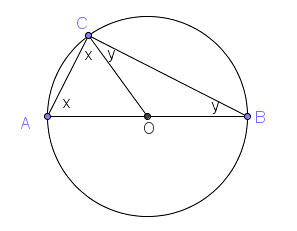# Thales’ Theorem

Thales was a Greek mathematician who lived in 654-625 BC. He was considered by many as the first mathematician.   One of his famous achievements was devising the first formal geometric proof. The theorem he proved is what is now known as Thales’ Theorem.The Theorem

Thales’ Theorem states that if$A$,$B$, and$C$ are points on the circle, and$AB$ is a diameter, then angle$ACB$ a right angle.You should explore this by drawing circles and different sizes and drawing the triangle. You may also want to use a dynamic software.The Proof

The proof of Thales’ Theorem is quite easy. First, we draw line segment$OC$ to form two isosceles triangles$OCA$ and$BOC$. Now, the base of the isosceles triangles are congruent as indicated by$x$ and$y$ in the second figure. The sum of the interior angles of a triangle$ABC$ is$180^\circ$. So,$x + ( x + y) + y = 180^\circ$.

Simplifying, we have$2x + 2y = 180^\circ$

Dividing both sides by 2, we have$x + y = 90^\circ$

But$(x + y)$ is the measure of angle$C$.

Therefore, the measure of angle$C$ is$90^\circ$. And we are done.